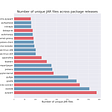# Optimal Secondary RNA Alignment and Nussinov’s Algorithm

Note: Before reading this article, read to understand primary sequence alignment and dynamic programming; here, we extend our analysis to secondary RNA alignment through Nussinov’s Algorithm.

# Terminology

## RNA Terms

• RNA: ribonucleic acid comprised of the 5-carbon sugar, ribose, which is attached to an organic base
• Uracil: a non-methylated nucleotide base in place of thymine which provides flexibility to RNA
• Riboswitches: a regulatory segment of mRNA molecule that binds a small molecule
• microRNAs: novel non-protein layers of gene regulation
• piRNA: largest class of small non-coding RNA molecules in animals — silencing of transposons, epigenetic modifications, and post-transcriptional gene silencing
• lncRNAs: long transcripts produced operating functionally as RNAs — not translated to proteins

## RNA Structure

RNA structure is comprised of three different levels: primary, secondary, and tertiary.

• Primary: sequence in which the bases (U, A, C, G) are aligned
• Secondary: 2-D analysis of hydrogen bonds between different parts of RNA — double-strand
• Tertiary: complete 3-D structure of RNA — “how string bends where it twists”

## Secondary Structure

Let a secondary structure be a vertex-labeled graph on n vertices with an adjacency matrix A. Let A (i, j) be the adjacency between the ith and jth base pair in a transcriptome.

Here are some assumptions:

• A continuous backbone is defined when A(i, i+1) = 1 for all i from 1 to n.
• For each i from 1 to n, there is at most one A(i, j) = 1 where j <i-1. This means a base only forms a pair with one other at the time.
• We ignore pseudo knots by saying if A(i,j)=A(k,l)=1 and i < k < j, then i < l < j.

# Nussinov’s Algorithm

## Goal

Our goal through this analysis is to predict the secondary structure of RNA, given its primary structure or sequence. Using dynamic programming, this can be found by creating a structure with the least free energy, where free energy is defined arbitrarily by a model.

By convention, negative free energy is stabilizing, while positive free energy is non-stabilizing. Note dynamic programming can be used for the following reasons:

• The scoring scheme of the model follows an additive property.
• Psuedoknots were not allowed, which means the RNA can be split into two smaller ones that are independent of one another.

Thus, mathematically, we want to find a matrix E(i,j) where we can calculate the minimum free energy for subsequence i to j.

## Algorithm — Initialization

A simple initialization matrix can be seen here where the diagonal is initialized to 0, and where complementary base pairings are stored as -1, while noncomplementary base pairings are stored as 0.

## Algorithm — DP

The intuition is as follows:

• With a subsequence from i to j, there is either no edge connecting the ith base (unpaired) or there is some edge connecting the ith based to the kth base where i < k ≤ j.
• If the edge is unpaired, the energy of subsequence E(i,j) reduces to the energy of a subsequence E(i+1, j).
• If the edge is paired, then the energy of subsequence E(i,j) becomes E(i, k-1) + E(k+1, j) + the energy contribution of the i,k pairing.

The model then backtracks through the entire matrix to find the optimal free energy alignment.

Let K be the traceback matrix. If K(i,j) = 0 then the model backtracks to K(i+1, j) as mentioned above. If K(i,j)=1, then the model backtracks to both K(i+1, K(i,j)-1) and K(K(i,j) +1, j). This continues until the optimal structure is found.

## Applications

By being able to calculate the optimal secondary alignment state, bioinformaticians will better be able to understand the method to which a specific RNA strand might fold itself, giving better insight into its functions for future analysis.

# TL;DR

• Nussinov’s algorithm is a method to calculate the secondary sequence of RNA from a primary sequence using dynamic programming to calculate the minimum free energy.
• Nussinov’s algorithm only works in specific conditions, such as without pseudoknots and with small numbers of base pairs.

If you want to talk more, schedule a meeting: ! If you’re interested in connecting, follow me on , , and .

--

--

--

Love podcasts or audiobooks? Learn on the go with our new app.

## Sentiment Analysis of Tweets on the Ukrainian Crisis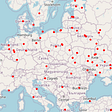## The Power of Multi-Task Learning for Bioinformatics (Potentially)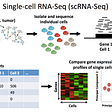## Optimizing Hyperparameters the right Way## On Trusting the Model## PySpark UDFs, Spark-NLP, and scrapping HTML files on spark clusters — a complete ETL process for…## Conditional GAN using PyTorch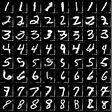## A Gentle Introduction To Genetic Algorithms## Jail or Bail?## More from Medium## Data Scientist: Which area of specialization to take?## How Useful is EDA in a Data Science or Machine Learning Project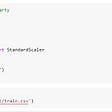## Snake JARs, Part III: Data Science Sssssss-security